# Some plots to go with group based trajectory models in R

On my prior post on estimating group based trajectory models in R using the crimCV package I received a comment asking about how to plot the trajectories. The crimCV model object has a base plot object, but here I show how to extract those model predictions as well as some other functions. Many of these plots are illustrated in my paper for crime trajectories at micro places in Albany (forthcoming in the Journal of Quantitative Criminology). First we are going to load the `crimCV` and the `ggplot2` package, and then I have a set of four helper functions which I will describe in more detail in a minute. So run this R code first.

``````library(crimCV)
library(ggplot2)

long_traj <- function(model,data){
df <- data.frame(data)
vars <- names(df)
prob <- model['gwt'] #posterior probabilities
df\$GMax <- apply(prob\$gwt,1,which.max) #which group # is the max
df\$PMax <- apply(prob\$gwt,1,max)       #probability in max group
df\$Ord <- 1:dim(df)                 #Order of the original data
prob <- data.frame(prob\$gwt)
names(prob) <- paste0("G",1:dim(prob)) #Group probabilities are G1, G2, etc.
longD <- reshape(data.frame(df,prob), varying = vars, v.names = "y",
timevar = "x", times = 1:length(vars),
direction = "long") #Reshape to long format, time is x, y is original count data
return(longD)                        #GMax is the classified group, PMax is the probability in that group
}

weighted_means <- function(model,long_data){
G_names <- paste0("G",1:model\$ng)
G <- long_data[,G_names]
W <- G*long_data\$y                                    #Multiple weights by original count var
Agg <- aggregate(W,by=list(x=long_data\$x),FUN="sum")  #then sum those products
mass <- colSums(model\$gwt)                            #to get average divide by total mass of the weight
for (i in 1:model\$ng){
Agg[,i+1] <- Agg[,i+1]/mass[i]
}
long_weight <- reshape(Agg, varying=G_names, v.names="w_mean",
timevar = "Group", times = 1:model\$ng,
direction = "long")           #reshape to long
return(long_weight)
}

pred_means <- function(model){
prob <- model\$prob               #these are the model predicted means
Xb <- model\$X %*% model\$beta     #see getAnywhere(plot.dmZIPt), near copy
lambda <- exp(Xb)                #just returns data frame in long format
p <- exp(-model\$tau * t(Xb))
p <- t(p)
p <- p/(1 + p)
mu <- (1 - p) * lambda
t <- 1:nrow(mu)
myDF <- data.frame(x=t,mu)
long_pred <- reshape(myDF, varying=paste0("X",1:model\$ng), v.names="pred_mean",
timevar = "Group", times = 1:model\$ng, direction = "long")
return(long_pred)
}

#Note, if you estimate a ZIP model instead of the ZIP-tau model
#use this function instead of pred_means
pred_means_Nt <- function(model){
prob <- model\$prob               #these are the model predicted means
Xb <- model\$X %*% model\$beta     #see getAnywhere(plot.dmZIP), near copy
lambda <- exp(Xb)                #just returns data frame in long format
Zg <- model\$Z %*% model\$gamma
p <- exp(Zg)
p <- p/(1 + p)
mu <- (1 - p) * lambda
t <- 1:nrow(mu)
myDF <- data.frame(x=t,mu)
long_pred <- reshape(myDF, varying=paste0("X",1:model\$ng), v.names="pred_mean",
timevar = "Group", times = 1:model\$ng, direction = "long")
return(long_pred)
}

occ <- function(long_data){
subdata <- subset(long_data,x==1)
agg <- aggregate(subdata\$PMax,by=list(group=subdata\$GMax),FUN="mean")
names(agg) <- "AvePP" #average posterior probabilites
agg\$Freq <- as.data.frame(table(subdata\$GMax))[,2]
n <- agg\$AvePP/(1 - agg\$AvePP)
p <- agg\$Freq/sum(agg\$Freq)
d <- p/(1-p)
agg\$OCC <- n/d #odds of correct classification
agg\$ClassProp <- p #observed classification proportion
#predicted classification proportion
agg\$PredProp <- colSums(as.matrix(subdata[,grep("^[G][0-9]", names(subdata), value=TRUE)]))/sum(agg\$Freq)
#Jeff Ward said I should be using PredProb instead of Class prop for OCC
agg\$occ_pp <- n/ (agg\$PredProp/(1-agg\$PredProp))
return(agg)
}
``````

Now we can just use the data in the crimCV package to run through an example of a few different types of plots. First lets load in the `TO1adj` data, estimate the group based model, and make our base plot.

``````data(TO1adj)
plot(out1)``````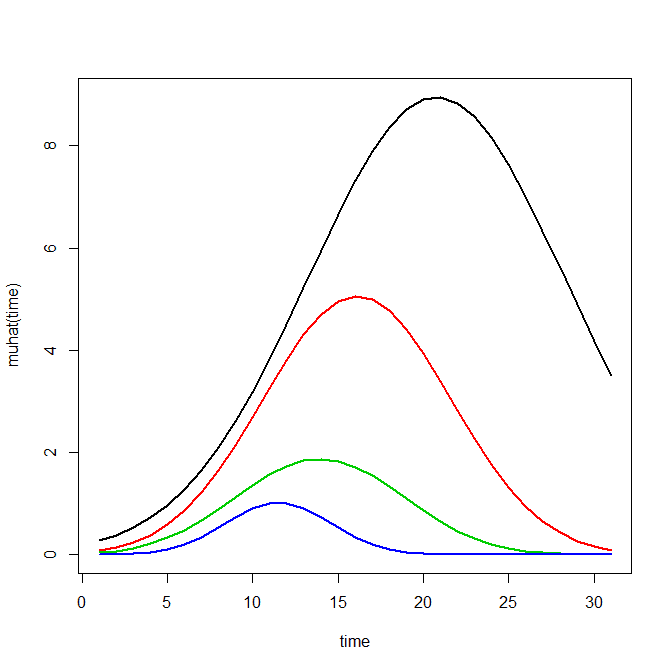Now most effort seems to be spent on using model selection criteria to pick the number of groups, what may be called relative model comparisons. Once you pick the number of groups though, you should still be concerned with how well the model replicates the data at hand, e.g. absolute model comparisons. The graphs that follow help assess this. First we will use our helper functions to make three new objects. The first function, `long_traj`, takes the original model object, `out1`, as well as the original matrix data used to estimate the model, `TO1adj`. The second function, `weighted_means`, takes the original model object and then the newly created long_data `longD`. The third function, `pred_means`, just takes the model output and generates a data frame in wide format for plotting (it is the same underlying code for plotting the model).

``````longD <- long_traj(model=out1,data=TO1adj)
x <- weighted_means(model=out1,long_data=longD)
pred <- pred_means(model=out1)``````

We can subsequently use the long data `longD` to plot the individual trajectories faceted by their assigned groups. I have an answer on cross validated that shows how effective this small multiple design idea can be to help disentangle complicated plots.

``````#plot of individual trajectories in small multiples by group
p <- ggplot(data=longD, aes(x=x,y=y,group=Ord)) + geom_line(alpha = 0.1) + facet_wrap(~GMax)
p ``````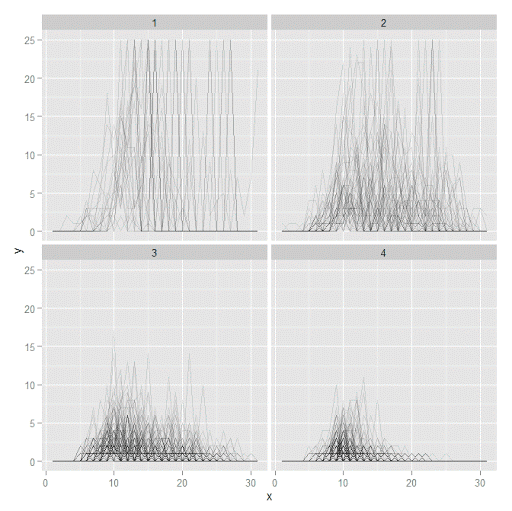Plotting the individual trajectories can show how well they fit the predicted model, as well as if there are any outliers. You could get more fancy with jittering (helpful since there is so much overlap in the low counts) but just plotting with a high transparency helps quite abit. This second graph plots the predicted means along with the weighted means. What the `weighted_means` function does is use the posterior probabilities of groups, and then calculates the observed group averages per time point using the posterior probabilities as the weights.

``````#plot of predicted values + weighted means
p2 <- ggplot() + geom_line(data=pred, aes(x=x,y=pred_mean,col=as.factor(Group))) +
geom_line(data=x, aes(x=x,y=w_mean,col=as.factor(Group))) +
geom_point(data=x, aes(x=x,y=w_mean,col=as.factor(Group)))
p2``````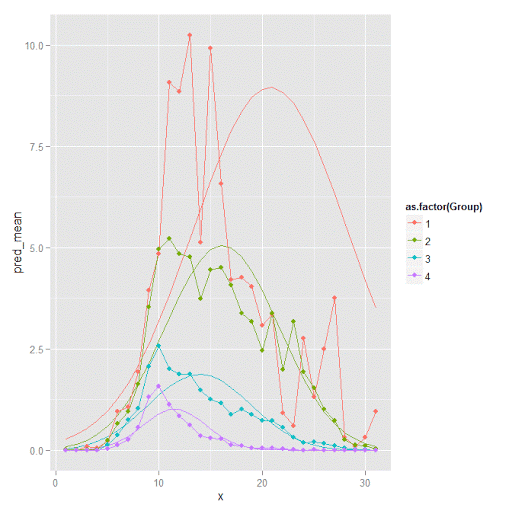Here you can see that the estimated trajectories are not a very good fit to the data. Pretty much eash series has a peak before the predicted curve, and all of the series except for 2 don’t look like very good candidates for polynomial curves.

It ends up that often the weighted means are very nearly equivalent to the unweighted means (just aggregating means based on the classified group). In this example the predicted values are a colored line, the weighted means are a colored line with superimposed points, and the non-weighted means are just a black line.

``````#predictions, weighted means, and non-weighted means
nonw_means <- aggregate(longD\$y,by=list(Group=longD\$GMax,x=longD\$x),FUN="mean")
names(nonw_means) <- "y"

p3 <- p2 + geom_line(data=nonw_means, aes(x=x,y=y), col='black') + facet_wrap(~Group)
p3``````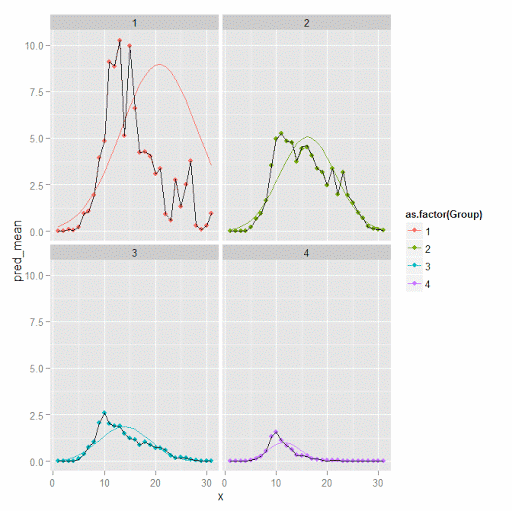You can see the non-weighted means are almost exactly the same as the weighted ones. For group 3 you typically need to go to the hundredths to see a difference.

``````#check out how close
nonw_means[nonw_means\$Group==3,'y'] -  x[x\$Group==3,'w_mean']``````

You can subsequently superimpose the predicted group means over the individual trajectories as well.

``````#superimpose predicted over ind trajectories
pred\$GMax <- pred\$Group
p4 <- ggplot() + geom_line(data=pred, aes(x=x,y=pred_mean), col='red') +
geom_line(data=longD, aes(x=x,y=y,group=Ord), alpha = 0.1) + facet_wrap(~GMax)
p4``````Two types of absolute fit measures I’ve seen advocated in the past are the average maximum posterior probability per group and the odds of correct classification. The `occ` function calculates these numbers given two vectors (one of the max probabilities and the other of the group classifications). We can get this info from our long data by just selecting a subset from one time period. Here the output at the console shows that we have quite large average posterior probabilities as well as high odds of correct classification. (Also updated to included the observed classified proportions and the predicted proportions based on the posterior probabilities. Again, these all show very good model fit.) Update: Jeff Ward sent me a note saying I should be using the predicted proportion in each group for the occ calculation, not the assigned proportion based on the max. post. prob. So I have updated to include the occ_pp column for this, but left the old occ column in as a paper trail of my mistake.

``````occ(longD)
#  group     AvePP Freq        OCC  ClassProp   PredProp     occ_pp
#1     1 0.9880945   23 1281.00444 0.06084656 0.06298397 1234.71607
#2     2 0.9522450   35  195.41430 0.09259259 0.09005342  201.48650
#3     3 0.9567524   94   66.83877 0.24867725 0.24936266   66.59424
#4     4 0.9844708  226   42.63727 0.59788360 0.59759995   42.68760
``````

A plot to accompany this though is a jittered dot plot showing the maximum posterior probability per group. You can here that groups 3 and 4 are more fuzzy, whereas 1 and 2 mostly have very high probabilities of group assignment.

``````#plot of maximum posterior probabilities
subD <- longD[x==1,]
p5 <- ggplot(data=subD, aes(x=as.factor(GMax),y=PMax)) + geom_point(position = "jitter", alpha = 0.2)
p5``````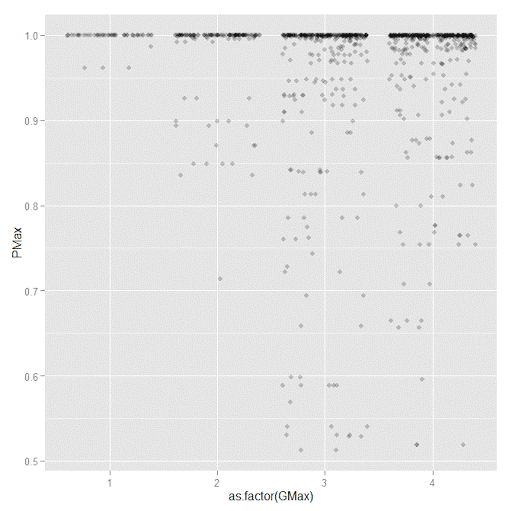Remember that these latent class models are fuzzy classifiers. That is each point has a probability of belonging to each group. A scatterplot matrix of the individual probabilities will show how well the groups are separated. Perfect separation into groups will result in points hugging along the border of the graph, and points in the middle suggest ambiguity in the class assignment. You can see here that each group closer in number has more probability swapping between them.

``````#scatterplot matrix
library(GGally)
sm <- ggpairs(data=subD, columns=4:7)
sm``````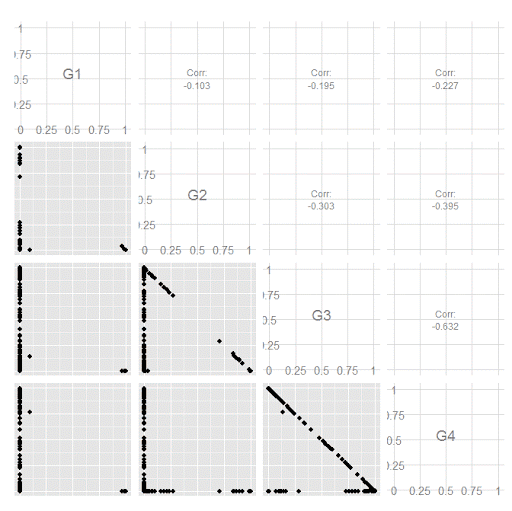And the last time series plot I have used previously is a stacked area chart.

``````#stacked area chart
nonw_sum <- aggregate(longD\$y,by=list(Group=longD\$GMax,x=longD\$x),FUN="sum")
names(nonw_sum) <- "y"
p6 <- ggplot(data=nonw_sum, aes(x=x,y=y,fill=as.factor(Group))) + geom_area(position='stack')
p6``````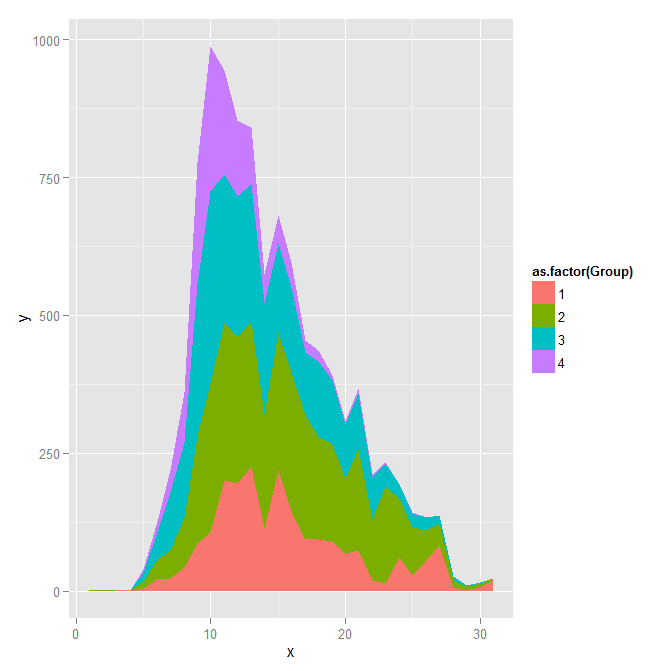I will have to put another post in the queue to talk about the spatial point pattern tests I used in that trajectory paper for the future as well.

# Custom square root scale (with negative values) in ggplot2 (R)

My prior rootogram post Jon Peck made the astute comment that rootograms typically are plotted on a square root scale. (Which should of been obvious to me given the name!) The reason for a square root scale for rootograms is visualization purposes, the square root scale gives more weight to values nearby 0 and shrinks values farther away from 0.

SPSS can not have negative values on a square root scale, but you can make a custom scale using ggplot2 and the scales package in R for this purpose. Here I just mainly replicated this short post by Paul Hiemstra.

So in R, first we load the `scales` and the `ggplot2` package, and then create our custom scale function. Obviously the square root of a negative value is not defined for real numbers, so what we do is make a custom square root function. The function simply takes the square root of the absolute value, and then multiplies by the sign of the original value. This function I name `S_sqrt` (for signed square root). We also make its inverse function, which is named `IS_sqrt`. Finally I make a third function, `S_sqrt_trans`, which is the one used by the scales package.

``````library(scales)
library(ggplot2)

S_sqrt <- function(x){sign(x)*sqrt(abs(x))}
IS_sqrt <- function(x){x^2*sign(x)}
S_sqrt_trans <- function() trans_new("S_sqrt",S_sqrt,IS_sqrt)``````

Here is a quick example data set in R to work with.

``````#rootogram example, see http://stats.stackexchange.com/q/140473/1036
MyText <- textConnection("
Dist Val1 Val2
1 0.03 0.04
2 0.12 0.15
3 0.45 0.50
4 0.30 0.24
5 0.09 0.04
6 0.05 0.02
7 0.01 0.01
")
MyData\$Hang <- MyData\$Val1 - MyData\$Val2``````

And now we can make our plots in `ggplot2`. First the linear scale, and second update our plot to the custom square root scale.

``````p <- ggplot(data=MyData, aes(x = as.factor(Dist), ymin=Hang, ymax=Val1)) +
geom_hline(aes(yintercept=0)) + geom_linerange(size=5) + theme_bw()
p

p2 <- p + scale_y_continuous(trans="S_sqrt",breaks=seq(-0.1,0.5,0.05), name="Density")
p2``````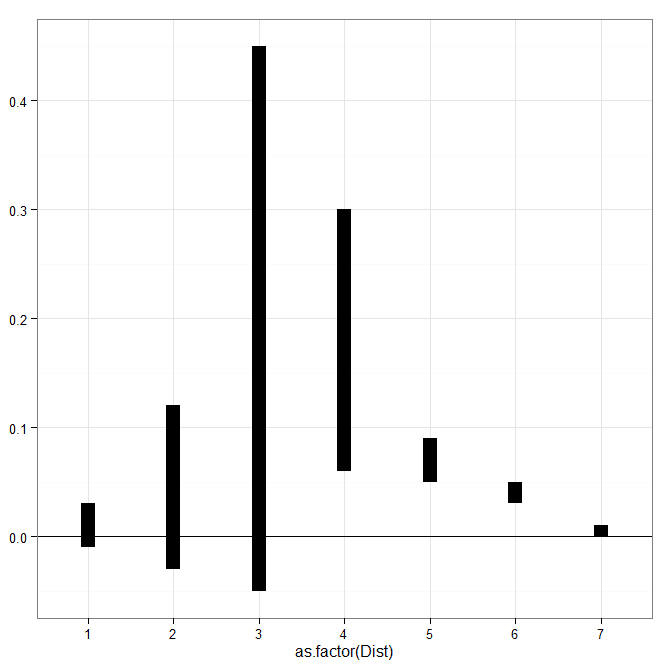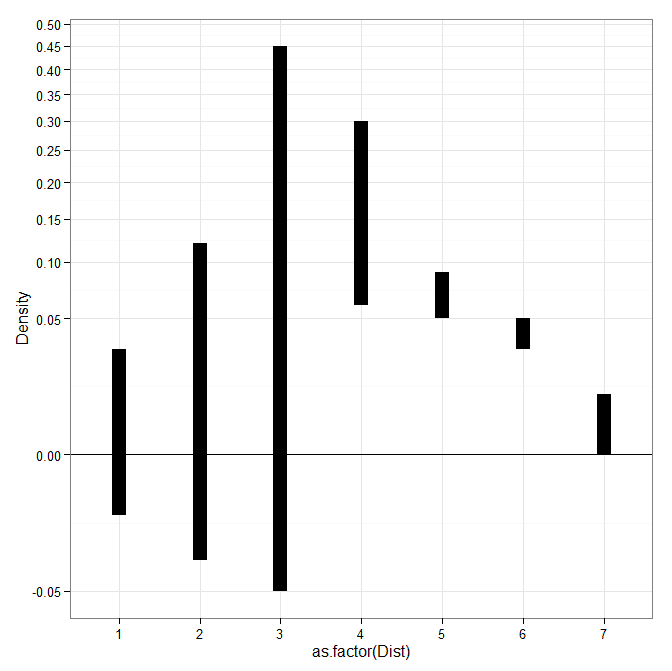# Jittered scatterplots with 0-1 data

Scatterplots with discrete variables and many observations take some touches beyond the defaults to make them useful. Consider the case of a categorical outcome that can only take two values, 0 and 1. What happens when we plot this data against a continuous covariate with my default chart template in SPSS?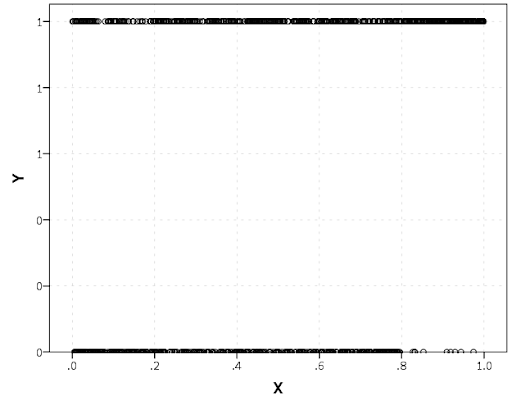Oh boy, that is not helpful. Here is the fake data I made and the `GGRAPH` code to make said chart.

``````*Inverse logit - see.
*https://andrewpwheeler.wordpress.com/2013/06/25/an-example-of-using-a-macro-to-make-a-custom-data-transformation-function-in-spss/.
DEFINE !INVLOGIT (!POSITIONAL  !ENCLOSE("(",")") )
1/(1 + EXP(-!1))
!ENDDEFINE.

SET SEED 5.
INPUT PROGRAM.
LOOP #i = 1 TO 1000.
COMPUTE X = RV.UNIFORM(0,1).
DO IF X <= 0.2.
COMPUTE YLin = -0.5 + 0.3*(X-0.1) - 4*((X-0.1)**2).
ELSE IF X > 0.2 AND X < 0.8.
COMPUTE YLin = 0 - 0.2*(X-0.5) + 2*((X-0.5)**2) - 4*((X-0.5)**3).
ELSE.
COMPUTE YLin = 3 + 3*(X - 0.9).
END IF.
COMPUTE #YLin = !INVLOGIT(YLin).
COMPUTE Y = RV.BERNOULLI(#YLin).
END CASE.
END LOOP.
END FILE.
END INPUT PROGRAM.
DATASET NAME NonLinLogit.
FORMATS Y (F1.0) X (F2.1).

*Original chart.
GGRAPH
/GRAPHDATASET NAME="graphdataset" VARIABLES=X Y
/GRAPHSPEC SOURCE=INLINE.
BEGIN GPL
SOURCE: s=userSource(id("graphdataset"))
DATA: X=col(source(s), name("X"))
DATA: Y=col(source(s), name("Y"))
GUIDE: axis(dim(1), label("X"))
GUIDE: axis(dim(2), label("Y"))
ELEMENT: point(position(X*Y))
END GPL.``````

So here we will do a few things to the chart to make it easier to interpret:

SPSS can jitter the points directly within `GGRAPH` code (see `point.jitter`), but here I jitter the data slightly myself a uniform amount. The extra aesthetic options for making points smaller and semi-transparent are at the end of the `ELEMENT` statement.

``````*Making a jittered chart.
COMPUTE YJitt = RV.UNIFORM(-0.04,0.04) + Y.
FORMATS Y YJitt (F1.0).
GGRAPH
/GRAPHDATASET NAME="graphdataset" VARIABLES=X Y YJitt
/GRAPHSPEC SOURCE=INLINE.
BEGIN GPL
SOURCE: s=userSource(id("graphdataset"))
DATA: X=col(source(s), name("X"))
DATA: Y=col(source(s), name("Y"))
DATA: YJitt=col(source(s), name("YJitt"))
GUIDE: axis(dim(1), label("X"))
GUIDE: axis(dim(2), label("Y"), delta(1), start(0))
SCALE: linear(dim(2), min(-0.05), max(1.05))
ELEMENT: point(position(X*YJitt), size(size."3"),
transparency.exterior(transparency."0.7"))
END GPL.``````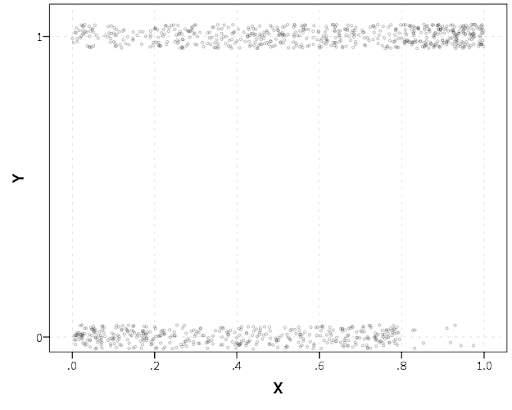If I made the Y axis categorical I would need to use `point.jitter` in the inline GPL code because SPSS will always force the categories to the same spot on the axis. But since I draw the Y axis as continuous here I can do the jittering myself.

A useful tool for exploratory data analysis is to add a smoothing term to plot – a local estimate of the mean at different locations of the X-axis. No binning necessary, here is an example using loess right within the `GGRAPH` call. The red line is the smoother, and the blue line is the actual proportion I generated the fake data from. It does a pretty good job of identifying the discontinuity at 0.8, but the change points earlier are not visible. Loess was originally meant for continuous data, but for exploratory analysis it works just fine on the 0-1 data here. See also `smooth.mean` for 0-1 data.

``````*Now adding in a smoother term.
COMPUTE ActualFunct = !INVLOGIT(YLin).
FORMATS Y YJitt ActualFunct (F2.1).
GGRAPH
/GRAPHDATASET NAME="graphdataset" VARIABLES=X Y YJitt ActualFunct
/GRAPHSPEC SOURCE=INLINE.
BEGIN GPL
SOURCE: s=userSource(id("graphdataset"))
DATA: X=col(source(s), name("X"))
DATA: Y=col(source(s), name("Y"))
DATA: YJitt=col(source(s), name("YJitt"))
DATA: ActualFunct=col(source(s), name("ActualFunct"))
GUIDE: axis(dim(1), label("X"))
GUIDE: axis(dim(2), label("Y"), delta(0.2), start(0))
SCALE: linear(dim(2), min(-0.05), max(1.05))
ELEMENT: point(position(X*YJitt), size(size."3"),
transparency.exterior(transparency."0.7"))
ELEMENT: line(position(smooth.loess(X*Y, proportion(0.2))), color(color.red))
ELEMENT: line(position(X*ActualFunct), color(color.blue))
END GPL.``````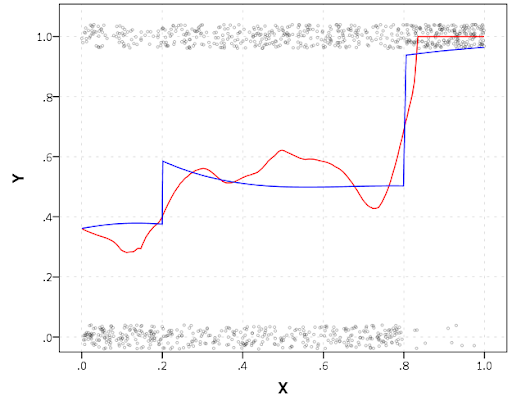SPSS’s default smoothing is alittle too smoothed for my taste, so I set the proportion of the X variable to use in estimating the mean within the `position` statement.

I wish SPSS had the ability to draw error bars around the smoothed means (you can draw them around the linear regression lines with quadratic or cubic polynomial terms, but not around the local estimates like `smooth.loess` or `smooth.mean`). I realize they are not well defined and rarely have coverage properties of typical regression estimators – but I rather have some idea about the error than no idea. Here is an example using the `ggplot2` library in R. Of course we can work the magic right within SPSS.

``````BEGIN PROGRAM R.
#Grab Data
casedata <- spssdata.GetDataFromSPSS(variables=c("Y","X"))
#ggplot smoothed version
library(ggplot2)
library(splines)
MyPlot <- ggplot(aes(x = X, y = Y), data = casedata) +
geom_jitter(position = position_jitter(height = .04, width = 0), alpha = 0.1, size = 2) +
stat_smooth(method="glm", family="binomial", formula = y ~ ns(x,5))
MyPlot
END PROGRAM.``````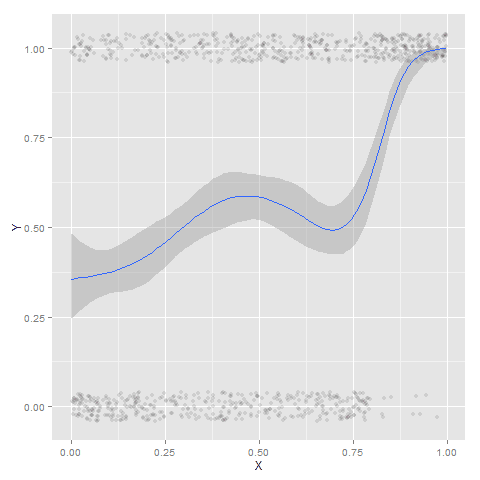To accomplish the same thing in SPSS you can estimate restricted cubic splines and then use any applicable regression procedure (e.g. `LOGISTIC`, `GENLIN`) and save the predicted values and confidence intervals. It is pretty easy to call the R code though!

I haven’t explored the automatic linear modelling, so let me know in the comments if there is a simply way right in SPSS to get explore such non-linear predictions.